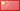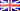中文版ENGLISH
How to choose a Power Inverter and Storage Battery?
1. You can choose Inverter in this way:

Most of the electronic products are signed out their rated power, and the rated power should be less than the rated power of the power inverter.

The method of how to estimate the power(W): the total of load power should be equal to the rated power(W) of the power inverter. For example, you use two computers(250W*2), a bulb(60W), a TV(200W), a fan(180W) at the same time, their total power is 250W*2+60W+200W+180W=940W, so you need a rated power 1000W power inverter with battery charge.

2. You can choose storage battery in this way:

You must choose storage battery that accord with DC input voltage. And it can supply enough working current(not less than the load input DC current of the power inverter with battery charge). Such as a power inverter with battery charge of 12V-22V-2000W, you should choose a storage battery that more than 200AH.

Rated power(W) (VA=W)
—————————————  *1.2 = ？A (A or AH)
Input voltage(such as 12V)

You can calculate that the storage attery's used time by this formula:

Battery capability (AH)
——————————————————  * 80% * 90% = Using time (Hour)
Load General Power / DC Input Voltage

Attention: The use time of storage battery is determined by the quality of storage battery and the time when you bought it.